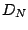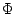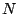Next: Fourier analysis of non-periodic Up: Properties of Fourier transforms Previous: Shifts and phase changes   Contents   Index

Fourier transform of a sinusoid

We can use the phase shift formula above to find the Fourier transform of any complex sinusoidwith frequency, simply by settingin the formula and using the Fourier transform for DC:whereis the Dirichlet kernel andis an ugly phase term:If the sinusoid's frequencyis an integer multiple of the fundamental frequency, the Dirichlet kernel is shifted to the left or right by an integer. In this case the zero crossings of the Dirichlet kernel line up with integer values of, so that only one partial is nonzero. This is pictured in Figure 9.3 (part a).Part (b) shows the result when the frequencyfalls halfway between two integers. The partials have amplitudes falling off roughly asin both directions, measured from the actual frequency. That the energy should be spread over many partials, when after all we started with a single sinusoid, might seem surprising at first. However, as shown in Figure 9.4, the signal repeats at a periodwhich disagrees with the frequency of the sinusoid. As a result there is a discontinuity at the beginning of each period, and energy is flung over a wide range of frequencies.Next: Fourier analysis of non-periodic Up: Properties of Fourier transforms Previous: Shifts and phase changes   Contents   Index
Miller Puckette 2006-12-30